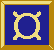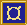# ¤ mapstateF

Abstract fudgets: stateful

## Types

`mapstateF :: (t -> a -> (t, [b])) -> t -> F a b`

## Synopsis

`mapstateF stf s0`

## Description

`mapstateF` creates abstract fudgets with internal state. A state transition function determines its behaviour.

### Input

will become arguments to `stf`.

### Output

results from applications of `stf`.

## Arguments

`stf :: t -> a -> (t, [b])`
a state transition function. Whenever an input message appears, `stf` is applied to the message and the current state. The result from the application is a new internal state and a list of output messages.

`s0 :: t`
initial internal state.

## Example

` countF = mapstateF count 0 where count n _ = (n+1,[n+1])`

## Example

` sumF = mapstateF add 0 where add acc n = (acc+n,[acc+n])`

## Equalities

```  mapstateF stf s0 = absF (concatMapAccumlSP stf s0)
```